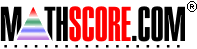Math Practice Online > free > lessons > Florida > 6th grade > Missing Factor

## Missing Factor

Given an equation, supply the missing factor.

 Sample Problems for Missing Factor Lesson for Missing Factor

### This topic aligns to the following state standards

Grade 4: Alg 1. Solves problems involving equations or simple inequalities using manipulatives, diagrams, or models, symbolic expressions, or written phrases.
Grade 5: Alg 1. Solves problems involving simple equations or inequalities using diagrams or models, symbolic expressions, or written phrases.

Copyright Accurate Learning Systems Corporation 2008.